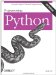# Section 23.5. Using Python Classes in C

### 23.5. Using Python Classes in C

In the previous chapter, we saw how to use C++ classes in Python by wrapping them with SWIG. But what about going the other wayusing Python classes from other languages? It turns out that this is really just a matter of applying interfaces already shown.

Recall that Python scripts generate class instance objects by calling class objects as though they were functions. To do it from C (or C++), simply follow the same steps: import a class from a module (or elsewhere), build an arguments tuple, and call it to generate an instance using the same C API tools you use to call Python functions. Once you've got an instance, you can fetch attributes and methods with the same tools you use to fetch globals out of a module.

To illustrate how this works in practice, Example 23-12 defines a simple Python class in a module that we can utilize from C.

##### Example 23-12. PP3E\Integrate\Embed\ApiClients\module.py

 ` # call this class from C to make objects class klass: def method(self, x, y): return "brave %s %s" % (x, y) # run me from C `

This is nearly as simple as it gets, but it's enough to illustrate the basics. As usual, make sure that this module is on your Python search path (e.g., in the current directory, or one listed on your PYTHONPATH setting), or else the import call to access it from C will fail, just as it would in a Python script. Now, here is how you might make use of this Python class from a Python program:

` ...\PP3E\Integrate\Embed\ApiClients\$ python >>> import module                                   # import the file >>> object = module.klass( )                       # make class instance >>> result = object.method('sir', 'robin')          # call class method >>> print result brave sir robin `

This is fairly easy in Python. You can do all of these operations in C too, but it takes a bit more code. The C file in Example 23-13 implements these steps by arranging calls to the appropriate Python API tools.

##### Example 23-13. PP3E\Integrate\Embed\ApiClients\objects-low.c

 ` #include #include main( ) { /* run objects with low-level calls */ char *arg1="sir", *arg2="robin", *cstr; PyObject *pmod, *pclass, *pargs, *pinst, *pmeth, *pres; /* instance = module.klass( ) */ Py_Initialize( ); pmod = PyImport_ImportModule("module"); /* fetch module */ pclass = PyObject_GetAttrString(pmod, "klass"); /* fetch module.class */ Py_DECREF(pmod); pargs = Py_BuildValue("( )"); pinst = PyEval_CallObject(pclass, pargs); /* call class( ) */ Py_DECREF(pclass); Py_DECREF(pargs); /* result = instance.method(x,y) */ pmeth = PyObject_GetAttrString(pinst, "method"); /* fetch bound method */ Py_DECREF(pinst); pargs = Py_BuildValue("(ss)", arg1, arg2); /* convert to Python */ pres = PyEval_CallObject(pmeth, pargs); /* call method(x,y) */ Py_DECREF(pmeth); Py_DECREF(pargs); PyArg_Parse(pres, "s", &cstr); /* convert to C */ printf("%s\n", cstr); Py_DECREF(pres); } `

Step through this source file for more details; it's mostly a matter of figuring out how you would accomplish the task in Python, and then calling equivalent C functions in the Python API. To build this source into a C executable program, run the makefile in the file's directory (it's analogous to makefiles we've already seen, so we'll omit it heresee the book's examples distribution package for a listing). After compiling, run it as you would any other C program:

` .../PP3E/Integrate/Embed/ApiClients\$ objects-low brave sir robin `

This output might seem anticlimactic, but it actually reflects the return values sent back to C by the class method in the file module.py. C did a lot of work to get this little string: it imported the module, fetched the class, made an instance, and fetched and called the instance method, performing data conversions and reference count management every step of the way. In return for all the work, C gets to use the techniques shown in this file to reuse any Python class.

Of course, this example would be more complex in practice. As mentioned earlier, you generally need to check the return value of every Python API call to make sure it didn't fail. The module import call in this C code, for instance, can fail easily if the module isn't on the search path; if you don't trap the NULL pointer result, your program will almost certainly crash when it tries to use the pointer (at least eventually). Example 23-14 is a recoding of Example 23-13 with full error-checking; it's big, but it's robust.

##### Example 23-14. PP3E\Integrate\Embed\ApiClients\objects-err-low.c

 ` #include #include #define error(msg) do { printf("%s\n", msg); exit(1); } while (1) main( ) { /* run objects with low-level calls and full error checking */ char *arg1="sir", *arg2="robin", *cstr; PyObject *pmod, *pclass, *pargs, *pinst, *pmeth, *pres; /* instance = module.klass( ) */ Py_Initialize( ); pmod = PyImport_ImportModule("module"); /* fetch module */ if (pmod == NULL) error("Can't load module"); pclass = PyObject_GetAttrString(pmod, "klass"); /* fetch module.class */ Py_DECREF(pmod); if (pclass == NULL) error("Can't get module.klass"); pargs = Py_BuildValue("( )"); if (pargs == NULL) { Py_DECREF(pclass); error("Can't build arguments list"); } pinst = PyEval_CallObject(pclass, pargs); /* call class( ) */ Py_DECREF(pclass); Py_DECREF(pargs); if (pinst == NULL) error("Error calling module.klass( )"); /* result = instance.method(x,y) */ pmeth = PyObject_GetAttrString(pinst, "method"); /* fetch bound method */ Py_DECREF(pinst); if (pmeth == NULL) error("Can't fetch klass.method"); pargs = Py_BuildValue("(ss)", arg1, arg2); /* convert to Python */ if (pargs == NULL) { Py_DECREF(pmeth); error("Can't build arguments list"); } pres = PyEval_CallObject(pmeth, pargs); /* call method(x,y) */ Py_DECREF(pmeth); Py_DECREF(pargs); if (pres == NULL) error("Error calling klass.method"); if (!PyArg_Parse(pres, "s", &cstr)) /* convert to C */ error("Can't convert klass.method result"); printf("%s\n", cstr); Py_DECREF(pres); } `Programming Python
ISBN: 0596009259
EAN: 2147483647
Year: 2004
Pages: 270
Authors: Mark Lutz